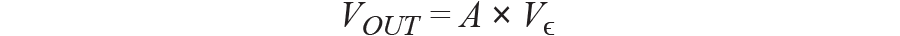ADALM1000 SMU Training Topic 2: Proportionality and Superposition

After the introduction of the SMU ADALM1000 in our Analog Dialogue December 2017 article, we want to start with the first of some small, basic measurements. You can find the previous ADALM1000 article here.

Now let’s get started with the second experiment.

Objective

The objective of this lab activity is to verify the proportionality and superposition theorems.

Background

In this activity, the proportionality and superposition theorems are examined by applying them to the circuits shown in the following figures.

1. The proportionality theorem states that the response of a circuit is proportional to the source acting on the circuit. This is also known as linearity. The proportionality constant A relates the input voltage to the output voltage as:The proportionality constant A is sometimes referred to as the gain of a circuit. For the circuit of Figure 2, the source voltage is VIN. The response VOUT is across the 4.7 kΩ resistor. The most important result of linearity is superposition.

2. The superposition theorem states that the response of a linear circuit with multiple independent sources, such as in Figure 3, can be obtained by adding the individual responses caused by the individual sources acting alone.

For an independent source acting alone, all other independent voltage sources in the circuit are replaced by short circuits and all other independent current sources are replaced by open circuits, as shown in Figure 4.

Materials

• Various resistors: 1 kΩ, 2.2 kΩ, and 4.7 kΩ.

Procedure

1. Verify the voltage division:

1. Construct the circuit of Figure 2. Using the Voltmeter tool, accurately measure VOUT for the three input voltages (using the ALM1000 fixed power supply voltages) as shown in Table 1. You should measure and record the actual fixed power supply voltages as well.

 VIN (volts) VOUT (volts) A (no units) 2.5 V 3.3 V 5.0 V
2. Calculate the value of A in each case using Equation 1.
3. Plot a graph with VIN on the x-axis and VOUT on the y-axis.
2. Verifying the superposition theorem:

1. Construct the circuit of Figure 3. Measure and record the voltage across the 4.7 kΩ resistor.
2. Construct the circuit of Figure 4. Measure and record the voltage across the 4.7 kΩ resistor.
3. Calculate the total response VOUT for the circuit of Figure 3 by adding the responses from Step 1a and Step 2b. Compare your calculated result to what you measured in Step 2a. Explain any differences.

Questions

1. Is the graph obtained a straight line? Compute the slope of the graph at any point and compare it to the value of K obtained from the measurements. Explain any differences.
2. For each of the three circuits you built for the superposition experiment, how well did the calculated and measured outputs compare? Explain any differences.

You can find the answers at the StudentZone blog.

Notes

As in all the ALM labs, we use the following terminology when referring to the connections to the ALM1000 connector and configuring the hardware. The green shaded rectangles indicate connections to the ADALM1000 analog I/O connector. The analog I/O channel pins are referred to as CA and CB. When configured to force voltage/measure current, –V is added as in CA-V or when configured to force current/measure voltage, –I is added as in CA-I. When a channel is configured in the high impedance mode to only measure voltage, –H is added as CA-H.

Scope traces are similarly referred to by channel and voltage/current, such as CA-V and CB-V for the voltage waveforms, and CA-I and CB-I for the current waveforms.

We are using the ALICE Rev 1.1 software for those examples here.

The ALICE desktop software provides the following functions:

• A 2-channel oscilloscope for time domain display and analysis of voltage and current waveforms.
• The 2-channel arbitrary waveform generator (AWG) controls.
• The X and Y display for plotting captured voltage and current vs. voltage and current data, as well as voltage waveform histograms.
• The 2-channel spectrum analyzer for frequency domain display and analysis of voltage waveforms.
• The Bode plotter and network analyzer with built-in sweep generator.
• An impedance analyzer for analyzing complex RLC networks and as an RLC meter and vector voltmeter.
• A dc ohmmeter measures unknown resistance with respect to known external resistor or known internal 50 Ω.
• Board self-calibration using the AD584 precision 2.5 V reference from the ADALP2000 analog parts kit.
• ALICE M1K voltmeter.
• ALICE M1K meter source.
• ALICE M1K desktop tool.